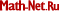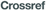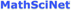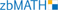# Semi-Invariant Form of Equilibrium Stability Criteria for Systems with One Cosymmetry

2019, Vol. 15, no. 4, pp.  525-531

Author(s): Kurakin L. G., Kurdoglyan A. V.

The systems of differential equations with one cosymmetry are considered . The ordinary object for such systems is a one-dimensional continuous family of equilibria. The stability spectrum changes along this family, but it necessarily contains zero. We consider the nondegeneracy condition, thus the boundary equilibria separate the family on linearly stable and instable areas. The stability of the boundary equilibria depends on nonlinear terms of the system.
The stability problem for the systems with one cosymmetry is studied in . The general problem is to apply the stability criteria one needs to compute coefficients of the model system. It is especially difficult if the system has a large dimension, while a number of critical variables may be small. A method for calculating coefficients is proposed in .
In this work the expressions for the known stability criteria are proposed in a form convenient for calculation. The explicit formulas of the coefficients of the model system are given in semi-invariant form. They are expressed using the generalized eigenvectors of the linear matrix and its conjugate matrix.
Keywords: stability, critical case, neutral manifold, cosymmetry, semi-invariant form
Citation: Kurakin L. G., Kurdoglyan A. V., Semi-Invariant Form of Equilibrium Stability Criteria for Systems with One Cosymmetry, Rus. J. Nonlin. Dyn., 2019, Vol. 15, no. 4, pp.  525-531
DOI:10.20537/nd190411

## References

 Yudovich, V. I., “Cosymmetry, Degeneration of Solutions of Operator Equations, and the Onset of Filtration Convection”, Math. Notes, 49:5–6 (1991), 540–545; Mat. Zametki, 49:5 (1991), 142–148 (Russian)Kurakin, L. G., “Critical Cases of Stability. Converse of the Implicit Function Theorem for Dynamical Systems with Cosymmetry”, Math. Notes, 63:3–4 (1998), 503–508; Mat. Zametki, 63:4 (1998), 572–578 (Russian)Kurakin, L. G. and Yudovich, V. I., “Semi-Invariant Form of Equilibrium Stability Criteria in Critical Cases”, J. Appl. Math. Mech., 50:5 (1986), 543–546; Prikl. Mat. Mekh., 50:5 (1986), 707–711 (Russian)Pliss, V. A., “A Reduction Principle in the Theory of Stability of Motion”, Izv. Akad. Nauk SSSR Ser. Mat., 28:6 (1964), 1297–1324 (Russian)Arnold, V. I., Additional Chapters of the Theory of Differential Equations, Nauka, Moscow, 1978, 304 pp. (Russian)Rumyantsev, V. V. and Oziraner, A. S., Motion Stability and Stabilization with Respect to Part of Variables, Nauka, Moscow, 1987, 256 pp. (Russian)